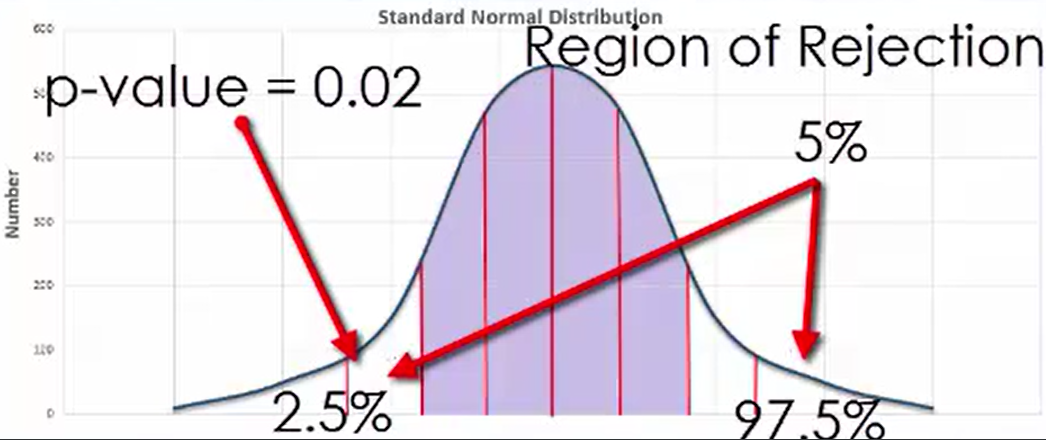+
Determining Significance Based on Probability
Interpret Inferential Statistics
0
of 0 possible points
1 Tutorials that teach Determining Significance Based on Probability

# Determining Significance Based on Probability

Author: Sophia Tutorial
##### Description:

Determine the relationship between probability and significance.

(more)

Sophia’s self-paced online courses are a great way to save time and money as you earn credits eligible for transfer to many different colleges and universities.*

No credit card required

37 Sophia partners guarantee credit transfer.

299 Institutions have accepted or given pre-approval for credit transfer.

* The American Council on Education's College Credit Recommendation Service (ACE Credit®) has evaluated and recommended college credit for 32 of Sophia’s online courses. Many different colleges and universities consider ACE CREDIT recommendations in determining the applicability to their course and degree programs.

Tutorial
what's covered
This lesson discusses determining significance based on probability. By the end of this lesson, you should be able to understand the relationship between the level of significance and the probability that an event occurs. This lesson covers:
1. Hypotheses and Probability
2. Examples
1. Resting Heart Rate and Exercise
2. Texts and Pets
3. Travel and Gas Prices

## 1. Hypotheses and Probability

In the experimental method, the null hypothesis states that there is not a cause-and-effect relationship between the two variables, whereas the alternative hypothesis states that there might be such a relationship. The results of an experiment are considered significant if they are unlikely to occur when the null hypothesis is true.

Probability is a means of establishing how likely an event or a result of an experiment is. A p-value is the probability that a result occurs in the event that the null hypothesis is actually true. Results of an experiment are considered significant if they are unlikely to occur in the event that the null hypothesis is true. Similarly, you could say that the results of an experiment lie in the region of rejection with an area of 5%, and such a result is significant when its p-value is less than the significance level of 5%.

## 2. Examples

2a. Resting Heart Rate and Exercise
Consider the relationship between how often a person engages in cardiovascular exercise and his or her resting heart rate. The null hypothesis for such an experiment would be that regardless of how often a person exercises, it would have no impact on resting heart rate. However, since there is a cause-and-effect relationship between the frequency of cardio exercise and resting heart rate, you would expect the results of such an experiment to be significant.

Suppose, in this case, the p-value turns out to be 0.02, or 2%. As you can see on the graph below, the combination of the two-tailed region works out to be 5% of the entire region under the normal distribution curve. The results of this specific test resulted in a p-value located at the point shown, which lies on the region of rejection since it is less than the 5% level of significance.2b. Texts and Pets
Look at the relationship that exists between how many text messages a person sends per day and the number of pets that they have. Let’s also suppose that you have a pre-established level of significance of 5%. In a case such as this, you would expect to fail to reject the null hypothesis, and as such, would not be surprised to arrive at a p-value of 35%. Such a value would indicate that the results of the experiment are not significant, because it is greater than 5%.

2c. Travel and Gas Prices
What about the case of the average price of gasoline and the average annual number of miles traveled on vacation by Americans? Suppose that, having conducted two experiments on these variables, we arrived at results that yielded p-values of 1.5%. This is clearly expected given the nature of the relationship that exists between the two variables, and as such, means that the results are significant.

summary
This lesson looked at the relationship between hypotheses and probability. Probability can be used to support or invalidate a null hypothesis. It is important to understand the relationship between the level of significance and the probability that an event occurs. To illustrate these relationships, you looked at several examples.

Source: This work is adapted from Sophia author Dan Laub.

Rating